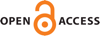Download this articleFor screen For printingRecent IssuesThe Journal About the Journal Editorial Board Editors’ Interests Subscriptions Submission Guidelines Submission Form Policies for Authors Ethics Statement ISSN: 1948-206X (e-only) ISSN: 2157-5045 (print) Author Index To Appear Other MSP JournalsA characterization of the Razak–Jacelon algebra

### Norio Nawata

Vol. 16 (2023), No. 8, 1799–1824##### Abstract

Combining Elliott, Gong, Lin and Niu’s result and Castillejos and Evington’s result, we see that if $A$ is a simple separable nuclear monotracial ${C}^{\ast }$-algebra, then $A\otimes \mathsc{𝒲}$ is isomorphic to $\mathsc{𝒲}$, where $\mathsc{𝒲}$ is the Razak–Jacelon algebra. In this paper, we give another proof of this. In particular, we show that if $\mathsc{𝒟}$ is a simple separable nuclear monotracial ${M}_{{2}^{\infty }}$-stable ${C}^{\ast }$-algebra which is $KK$-equivalent to $\left\{0\right\}$, then $\mathsc{𝒟}$ is isomorphic to $\mathsc{𝒲}$ without considering tracial approximations of ${C}^{\ast }$-algebras with finite nuclear dimension. Our proof is based on Matui and Sato’s technique, Schafhauser’s idea in his proof of the Tikuisis–White–Winter theorem and properties of Kirchberg’s central sequence ${C}^{\ast }$-algebra $F\left(\mathsc{𝒟}\right)$ of $\mathsc{𝒟}$. Note that some results for $F\left(\mathsc{𝒟}\right)$ are based on Elliott, Gong, Lin and Niu’s stable uniqueness theorem. Also, we characterize $\mathsc{𝒲}$ by using properties of $F\left(\mathsc{𝒲}\right)$. Indeed, we show that a simple separable nuclear monotracial ${C}^{\ast }$-algebra $D$ is isomorphic to $\mathsc{𝒲}$ if and only if $D$ satisfies the following properties:

1. For any $𝜃\in \left[0,1\right]$, there exists a projection $p$ in $F\left(D\right)$ such that ${\tau }_{D,\omega }\left(p\right)=𝜃$.

2. If $p$ and $q$ are projections in $F\left(D\right)$ such that $0<{\tau }_{D,\omega }\left(p\right)={\tau }_{D,\omega }\left(q\right)$, then $p$ is Murray–von Neumann equivalent to $q$.

3. There exists an injective homomorphism from $D$ to $\mathsc{𝒲}$.

##### Keywords
stably projectionless $\mathrm{C}^*$-algebra, Kirchberg's central sequence $\mathrm{C}^*$-algebra, $K\mkern-3mu K$-contractible $\mathrm{C}^*$-algebra, Razak–Jacelon algebra
##### Mathematical Subject Classification
Primary: 46L35
Secondary: 46L40, 46L80
##### Milestones